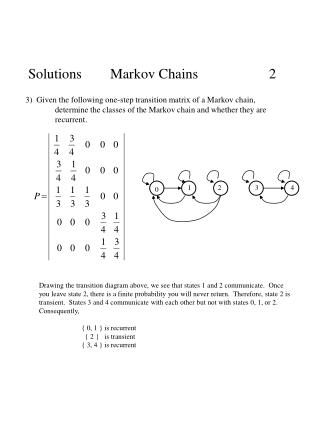Download PresentationSolutions Markov Chains 2

# Solutions Markov Chains 2 - PowerPoint PPT PresentationDownload Presentation## Solutions Markov Chains 2

- - - - - - - - - - - - - - - - - - - - - - - - - - - E N D - - - - - - - - - - - - - - - - - - - - - - - - - - -
##### Presentation Transcript

1. 1 2 3 4 0 Solutions Markov Chains 2 3) Given the following one-step transition matrix of a Markov chain, determine the classes of the Markov chain and whether they are recurrent. Drawing the transition diagram above, we see that states 1 and 2 communicate. Once you leave state 2, there is a finite probability you will never return. Therefore, state 2 is transient. States 3 and 4 communicate with each other but not with states 0, 1, or 2. Consequently, { 0, 1 } is recurrent { 2 } is transient { 3, 4 } is recurrent

2. Solutions Markov Chains 6 4) A computer is inspected at the end of every hour. It is found to be either working (up) or failed (down). If the computer is found to be up, the probability of its remaining up for the next hour is 0.90. It it is down, the computer is repaired, which may require more than one hour. Whenever, the computer is down (regardlewss of how long it has been down), the probability of its still being down 1 hour later is 0.35. a. Construct the one-step transition probability matrix. b. Find the expected first passage time from i to j for all i, j. Soln: Let, S = 0 if computer is down = 1 if computer is up Then, b. Find expected first passage times

3. Solutions Markov Chains 7 4) (cont.) b. Find expected first passage times

4. Solutions Markov Chains 8 4) (cont.) Alternative solution to b. (1) (2) (3) (4) Substituting (4) into (1) gives And from (4),

5. p = 0 . 13 0 p = 0 . 87 1 Solutions Markov Chains 9 4) (cont.) Alternative solution to b.

6. Solutions Markov Chains 10 5) A manufacturer has a machine that, when operational at the beginning of a day, has a probability of 0.1 of breaking down sometime during the day. When this happens, the repair is done the next day and completed at the end of that day. a. Formulate the evolution of the status of the machine as a 3 state Markov Chain. b. Fine the expected first passage times from i to j. c. Suppose the machine has gone 20 full days without a breakdown since the last repair was completed. How many days do we expect until the next breakdown/repair? Soln: a. Let, S = 0 if machine running at day’s end | running at start = 1 if machine down at day’s end | running at start = 2 if machine running at day’s end | down at start

7. Solutions Markov Chains 11 5) (cont.) a. S = 0 if machine running at day’s end | running at start = 1 if machine down at day’s end | running at start = 2 if machine running at day’s end | down at start Continuing in this fashion gives the one-step transition prob. b. 0 Note: This makes intuitive sense. If the machine has a 10% chance of failing on any given day, then the expected number of days between failures is 10, (m01 = 10).

8. Solutions Markov Chains 12 5) (cont.) 0 0 0

9. Solutions Markov Chains 13 5) (cont.) Back substituting for m02, m10, m11

10. Solutions Markov Chains 14 5) (cont.) c. Suppose the machine has gone 20 full days without a breakdown since the last repair was completed. How many days do we expect until the next breakdown/repair? If we read this as the expected number of days to breakdown since the last repair, we are asking for m21 in which case, If we read this as the expected number of days to breakdown and subsequent repair since the last repair, we are asking for m22. Again, this should make intuitive sense. A machine has a 10% chance of breaking down. Therefore, the expected time between failures is 10 days. Since it takes 1 day to repair, the time from repair to repair is 10+1 = 11 days.